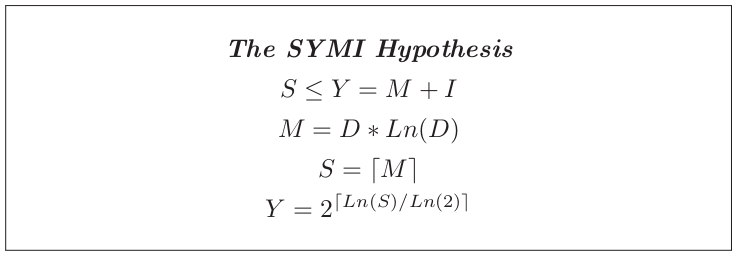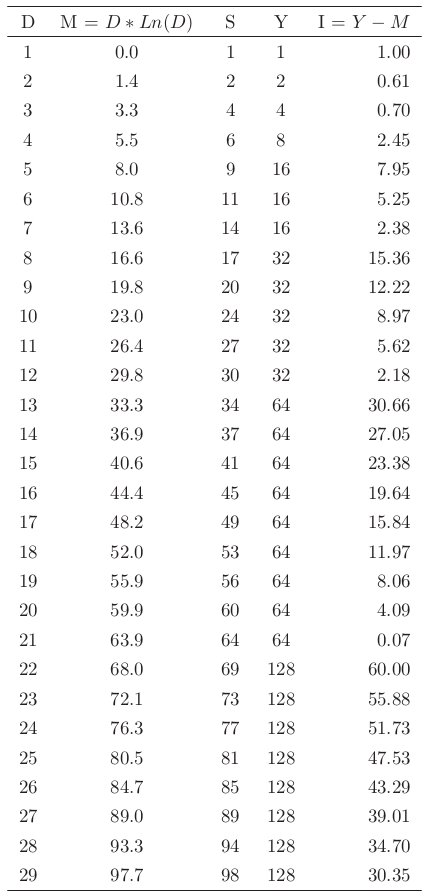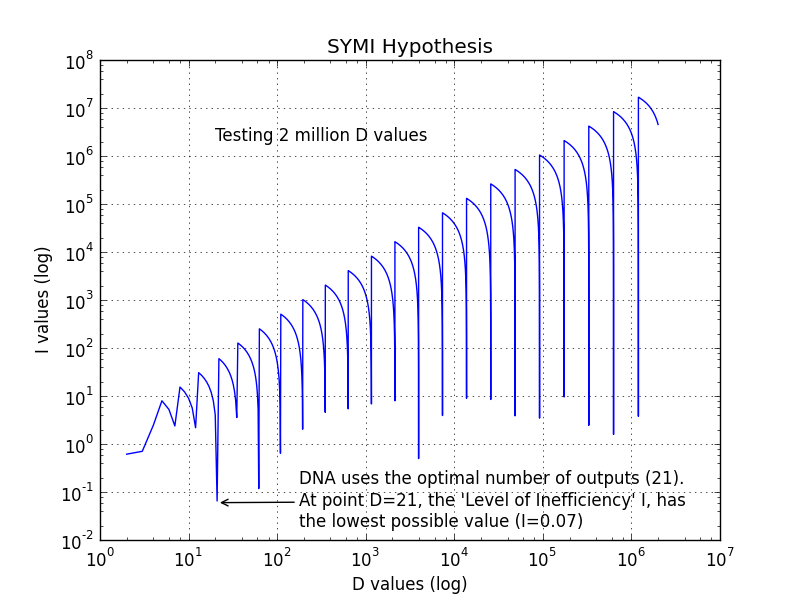# SYMI Hypothesis

We know that, in the process of protein synthesis, 64 unique inputs get translated to 21 unique outputs (20 amino acids plus the stop output).

Why is nature using 64 inputs and why only 21 outputs? This can be explained with the SYMI Hypothesis. I came up with this formula inspired by the work of Claude E. Shannon on Information Theory.Assume that a system, based on Y number of inputs can successfully carry D number of messages.

The formula M = D* ln(D) calculates the minimum number of inputs M required based on the number of messages D the system needs to carry.

I is the “Level of Inefficiency”. For those familiar with thermodynamics, think of this value as the heat generated when work is produced.

S is the minimum number of inputs required. Mathematically S is the ceiling of M. Y needs to be a power of 2, if we are assuming that the DNA is binary, and bigger or equal to S.

To reach optimal conditions, we clearly need to reduce the I value (Level of Inefficiency). This seems to be happening at the point where D=21 and S=64!

The table below shows the first 29 D values.I have mathematically checked the first 10 million D values and D=21 still gives the lowest possible I value.

Here is a graph of the first 2 million values of log(I) against log(D)To generate the graph above, just run this python script: https://github.com/binaryDNA/symi_hypothesis

Is it just a random coincidence that 64 inputs and 21 outputs have the lowest possible I value (Level of Inefficiency) out of 10 million possibilities? Unlikely.

The SYMI Hypothesis introduces a very interesting question, which I think will be better explained by mathematicians in the future. If it’s proven to be correct, it will show that nature has selected the 64 inputs /21 outputs combination because it’s the optimal way of safely transporting data with the help of an error correction mechanism.